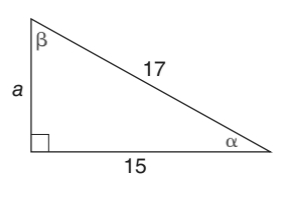Chapter 11.1, Problem 3E### Elementary Geometry for College St...

6th Edition
Daniel C. Alexander + 1 other
ISBN: 9781285195698

#### Solutions

Chapter
Section### Elementary Geometry for College St...

6th Edition
Daniel C. Alexander + 1 other
ISBN: 9781285195698
Textbook Problem
1 views

# In Exercises 1 to 6, find sin     α and sin     β for the triangle shown.To determine

To find:

The angle sinαandsinβ

Explanation

In a right angle triangle,

1) The side PQ, which is opposite to the right angle PRQ is called the hypotenuse.

(The hypotenuse is the longest side of the right triangle.)

2) The side RQ is called the adjacent side of angle θ.

3) The side PR is called the opposite side of angle θ.

Hence, the sine ratio for an acute angle is oppositehypotenuse

Given:

By using Pythagoras theorem, that is

c2=a2+b2

From, the diagram we have c=17andb=15

172=a2+152289=a2+225a

### Still sussing out bartleby?

Check out a sample textbook solution.

See a sample solution

#### The Solution to Your Study Problems

Bartleby provides explanations to thousands of textbook problems written by our experts, many with advanced degrees!

Get Started

#### In Exercises 49-62, find the indicated limit, if it exists. 49. limx1x21x1

Applied Calculus for the Managerial, Life, and Social Sciences: A Brief Approach

#### 56. Profit If the profit function for a product is given by Find .

Mathematical Applications for the Management, Life, and Social Sciences

#### Evaluate the integral. 36. /4/2cot3xdx

Single Variable Calculus: Early Transcendentals

#### For 0

Study Guide for Stewart's Multivariable Calculus, 8th